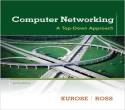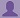SHARE
HELP

To start with, Sr2Jr’s first step is to reduce the expenses related to education. To achieve this goal Sr2Jr organized the textbook’s question and answers. Sr2Jr is community based and need your support to fill the question and answers. The question and answers posted will be available free of cost to all.# Computer Networking : A Top-down Approach

Authors:
James F. Kurose, Keith W. Ross
Exercise:
Problems
Chapter:
Transport Layer
Edition:
6
ISBN:
9780132856201
Question:
44
 Previous Next

###Question

Consider sending a large file from a host to another over a TCP connection that has no loss.

a. Suppose TCP uses AIMD for its congestion control without slow start. Assuming cwnd increases by 1 MSS every time a batch of ACKs is received and assuming approximately constant round-trip times, how long does it take for cwnd increase from 6 MSS to 12 MSS (assuming no loss events)?

b. What is the average throughout (in terms of MSS and RTT) for this connection up through time = 6 RTT?

###Answer

a)

Given data:

Assuming cwnd increases by 1 MSS every time a batch of ACKs is received and assuming approximately constant round-trip times.

Then transmission rate  of TCP is=w bytes/RTT

cwnd increases by 1 MSS if every batch of ACKs received.

The below steps are take for cwnd to increase from 6 MSS to 12 MSS:

• 1 RTTs to  to 7 MSS.
• 2 RTTs to 8 MSS.
• 3 RTTs to 9 MSS.
• 4 RTTs to 10 MSS.
• 5 RTTs to 11MSS.
• 6 RTTs to 12 MSS.

b)

Given data:

Connection up through time = 6 RTT

Average throughout (in terms of MSS and RTT) =(6+7+8+9+10+11)/6

=8.5 MSS/RTT

### Discussions

Post the discussion to improve the above solution.